## Pages

### Factoring Special Binomials

Here we will learn how to factor special binomials. We have seen some of the formulas in a previous section, however, we will be using them in a different manner here.

These formulas will be used as a template for factoring.Difference of SquaresSum of SquaresDifference of CubesSum of Cubes
The idea is to identify a and b and then plug in to the formulas above.

Factor:Step 1: Identify the special binomial.Step 2: Identify the a and the b in the formula.Step 3: Substitute into the appropriate formula.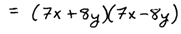Step 4: Check by multiplying.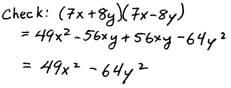The same steps can be used for the sum and difference of cubes formula.Review the perfect cubes up to 10. Many times the coefficients will give a clue as to what special binomial formula is to be used.

Factor.At this point we will look a bit more closely at the process. First identify the binomial that is to be factored.In this case, we have a sum of cubes were a = m and b = 2n. Next identify the appropriate formula and substitute into it.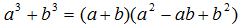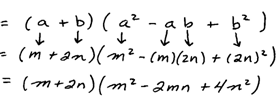After identifying the special binomial and determining the a and the b, it is just a matter of substituting into the appropriate formula.

Much of this can be done mentally, so it is sufficient to present your solution without the above steps.Rest assured that with much practice you will be able to jump straight to the answer too. The first step toward this ability, of course, is to memorize the formulas.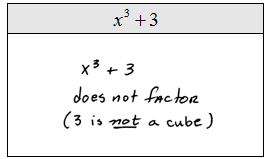As we have seen before, we will often run into polynomials with a GCF. It is important with special binomials to factor out the GCF first.

Factor: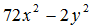As it stands, this binomial is not a difference of squares. Factor out the GCF and see that the remaining factor is a difference of squares.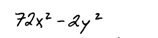The GCF is still part of the answer.

Factor.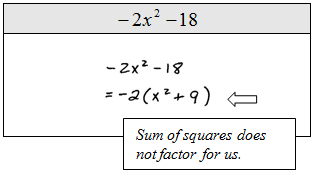Factoring binomials is a bit more complicated when larger exponents are involved. It is difficult to recognize that x^6, for example, is a perfect cube. We can think of x^6 = (x^2)^3  or the cube of x squared. Also, recall the rule of exponentsFactor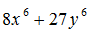Sum of cubes.It is not always necessary to show all the steps shown above. Ask your instructor what he or she wants to see in the way of steps when presenting your solutions in this case.

Factor.Look for factors that factor further. In other words, look to continue factoring until all factors are completely factored. Also, the trinomials that we obtain when using the sum and difference of cubes will not factor.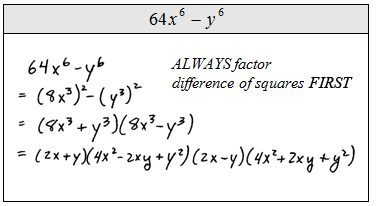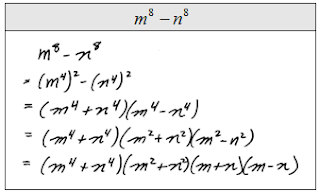If we are confronted with a polynomial that is both a difference of squares and a difference of cubes we must factor it as a difference of squares first. Doing this will ensure that we obtain a complete factorization.

Video Examples on YouTube: Factor the special binomials below.